# NCERT Solutions class-11 Maths Exercise 8.1## myCBSEguide App

CBSE, NCERT, JEE Main, NEET-UG, NDA, Exam Papers, Question Bank, NCERT Solutions, Exemplars, Revision Notes, Free Videos, MCQ Tests & more.

Exercise 8.1

Expand each of the expression in Exercises 1 to 5.

1.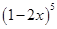Ans. Using Binomial Theorem,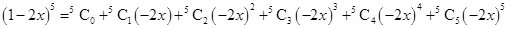=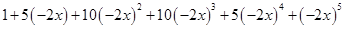=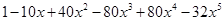2.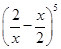Ans. Using Binomial Theorem,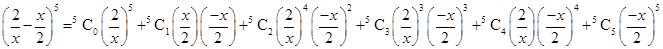=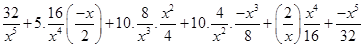=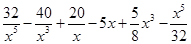3.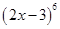Ans. Using Binomial Theorem,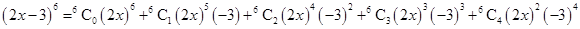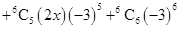=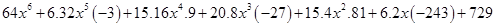=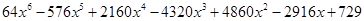4.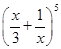Ans. Using Binomial Theorem,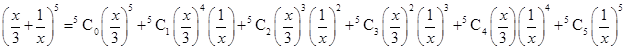=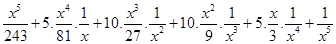=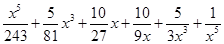5.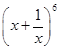Ans. Using Binomial Theorem,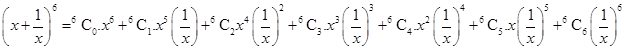=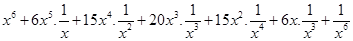=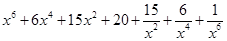Using binomial theorem evaluate each of the following:

6.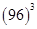Ans. Given: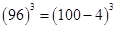Using Binomial Theorem,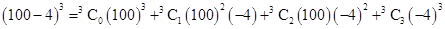=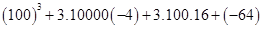= 1000000 – 120000 + 4800 – 64

= 1004800 – 120064 = 884736

7.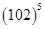Ans. Given: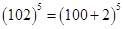Using Binomial Theorem,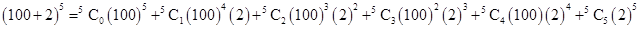=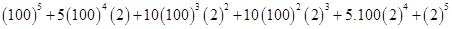= 10000000000 + 1000000000 + 40000000 + 800000 + 8000 + 32

= 11040808032

8.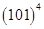Ans. Given: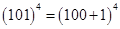Using Binomial Theorem,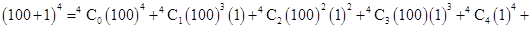=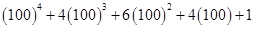= 100000000 + 4000000 + 60000 + 400 + 1

= 104060401

9.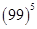Ans. Given: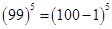Using Binomial Theorem,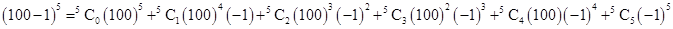=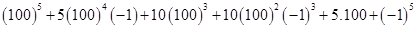= 10000000000 – 500000000 + 10000000 – 100000 + 500 – 1

= 9509900499

10. Using binomial theorem, indicate which number is larger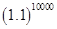or 1000.

Ans. Given: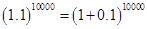Using Binomial Theorem,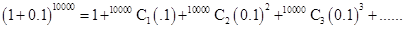= 1 + 10000 (0.1) + other positive numbers

= 1 + 1000 + other positive numbers

which is greater than 1000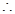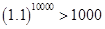11. Find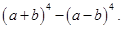Hence evaluate: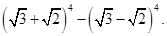Ans. Given: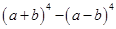Using Binomial Theorem,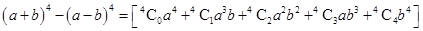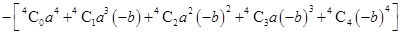=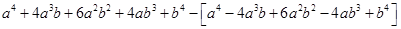=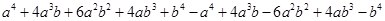=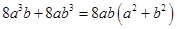Putting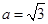and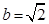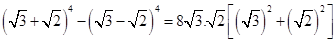=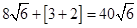12. Find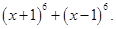Hence or otherwise evaluate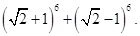Ans. Given: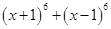Using Binomial Theorem,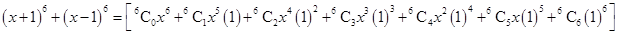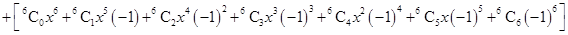=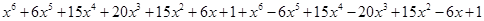=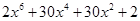=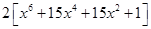Putting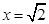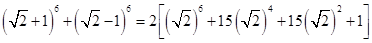=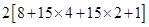= 2 [ 8 + 60 + 30 + 1] =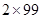= 198

13. Show that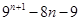is divisible by 64 whenever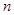is a positive integer.

Ans. Given: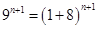Using Binomial Theorem,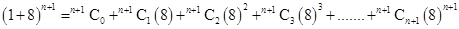=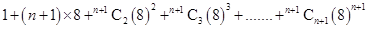=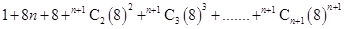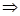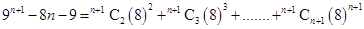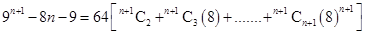which shows thatis divisible by 64.

14. Prove that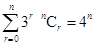Ans. L.H.S. =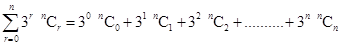=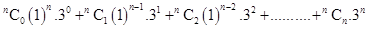=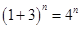= R.H.S.### 1 thought on “NCERT Solutions class-11 Maths Exercise 8.1”

1. Good solutions